# Bayes Data Science Hacks - Customizing in Depth with Matplotlib

There are a lot of good information sources for data scientists out there. Plenty of articles online will teach you regression with Sklearn, working with data frames in Pandas or basic neural network architectures in Tensorflow. In our new series "Bayes Data Science Hacks" we avoid the common topics and instead focus on small bits and pieces of code that you won't find anywhere else - but that will help you be successful in your data science career.

Today we look into using Matplotlib and Seaborn to create a visually more interesting plot. It always helps to know your standard libraries well enough to create visualisations quickly. Both Matplotlib and Seaborn provide you with some nice plotting options by default, but it's nice to be able to further customize them, if you need it.

Four our article about map selection advantage in Counter-Strike, for instance, we wanted to use something that was more creative and readable than a simple bar graph, but without spending too much time on it. One option would have been to draw it by hand - but that would have meant adjusting it manually every time the numbers change (and indeed, we had to adjust the numbers due to the delay between writing and publishing the article). This is what the final result looked like: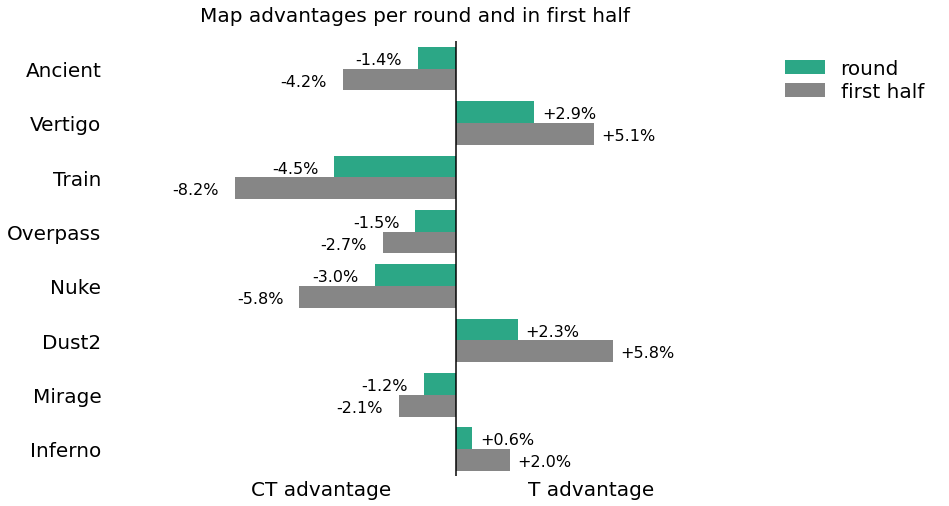Read on if you think it looks cool and want to learn how to recreate it in your own work!

Let’s start by doing our base imports and generating the data. In this example, we are using statistics about Counter-Strike maps: How often terrorists win rounds in the first half of each map and how often they end up winning the first half. Don't be confused by Ancient, at the time of writing the article, it was really new to the rotation, and the data we show here is in no way statistically significant. In the code snippet below we load some libraries, set plot colors, and then create a pandas DataFrame with the data.
1. import pandas as pd
2. import matplotlib.pyplot as plt
3. # since we like custom colors, we also immediately fix the colors for all our plots to be "Bayes":
4. plt.rcParams['axes.prop_cycle'] = plt.cycler(color=["#17BC90", "#868686"])
5. # generate the data
6. d = {'mapname': ['Ancient', 'Vertigo', 'Train', 'Overpass', 'Nuke', 'Dust2', 'Mirage', 'Inferno'], 'round win': [48.6, 52.9, 45.5, 48.5, 47.0, 52.3, 48.8, 50.6], '1st half win': [45.8, 55.1, 41.8, 47.3, 44.2, 55.8, 47.9, 52.0]}
7. df = pd.DataFrame(d)
The data frame looks like this: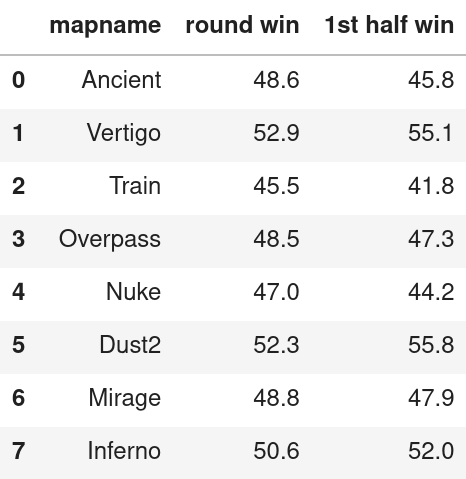In theory, we could plot this figure using the pandas builtin bar plot and be done with it:

1. # make a new figure
2. fig, ax = plt.subplots(figsize=(15,5))
3. # bar plot in the axes we just created. set_index is used to have the bar plot by map
4. df.set_index('mapname').plot.bar(ax=ax)
6. ax.set_title('Map advantages per round and in first half')
7. # make sure the map names are horizontal
8. plt.xticks(rotation=0)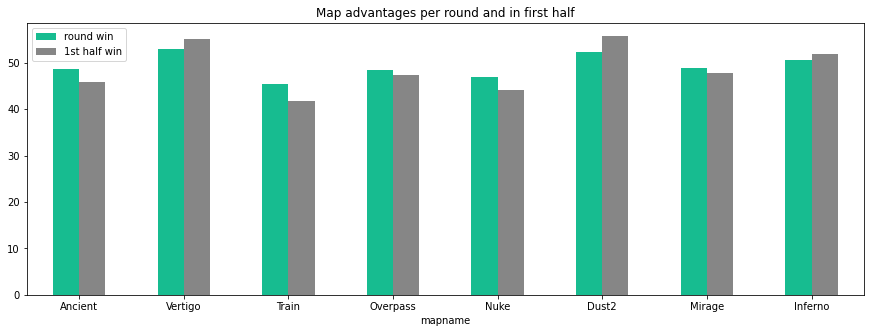For 90% of use cases, this plot is more than enough (might want to add a grid though). But few people will find it exciting, and exciting is what we aim for when we write “general target” articles. The first idea was to make the differences more prominent by showing the size of the advantage directly. This we can achieve by simply subtracting 50 from the values. We also switched to a horizontal bar plot because it looked nicer. We could have kept using Pandas but Seaborn is optimized for providing nice plots. Hence we change libraries. Since Seaborn does not per default let us plot two data frame columns at once, we need to first format our data:
1. # do some data manipulations - subtract 50 from the numerical columns, then add the mapname column back in as a column
2. to_plot = df.set_index('mapname')[['round win', '1st half win']].sub(50).reset_index()
3. to_plot = pd.melt(to_plot, id_vars=['mapname'])
The melt operation creates a data frame with only one 'value' column and a 'variable' column that tells you which variable it is. Some rows of the data frame we end up with are shown below. As you can see, there are now two rows for each map, one for the first half win and one for the round win value.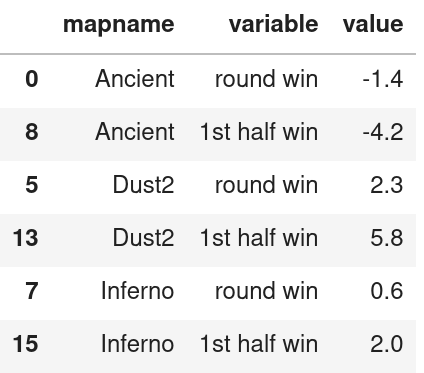Let us now plot this using seaborn:
1. import seaborn as sns
2. sns.barpot(data=to_plot, y='mapname', hue='variable', x='value')
3. ax.set_xlim([-13, 18])
4. ax.set_title('Map advantages per round and in first half                                  ', pad=20, fontsize = 20)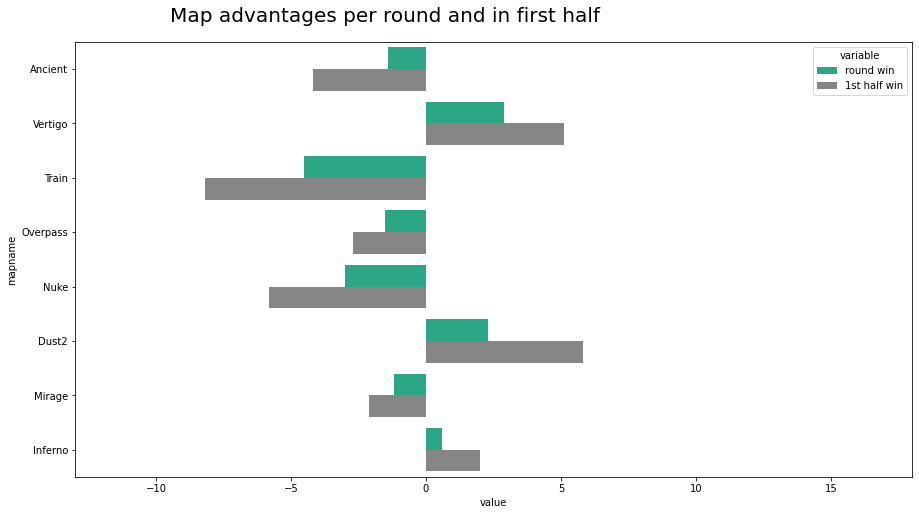This looks much more interesting already! But for someone unused to looking at graphs it's still hard to understand what is going on.

From here, we want to annotate the bars with the win percentage. No data science plotting package can do this directly - but we can use the annotate function of matplotlib to put text just about anywhere.

In the code below, we go through the patches - the individual elements that were plotted on our axis - in order to annotate them. Since our plot is centered around 0, they will have negative width for the counter-terrorist advantage and positive width for terrorist advantage. We want to plot the ct percentage to the left and terrorist percentage to the right of the bars. To find the exact location to put the text in, we use the x coordinate of the patch plus its width plus an offset. We do the same for the y coordinate. For the actual value, we use the width of the bar. We could just as well have used the corresponding value from the data set. The f'{}' notation works from python 3.6 on, if you are using an older version, you should use different string formatting. You might also consider upgrading to a more recent python version!
1. for i, p in enumerate(ax.patches):
2. if p.get_width() > 0: ax.annotate(f'+{abs(p.get_width()):.1f}%', (p.get_x()+p.get_width()+0.1, p.get_y() + p.get_height()+0.1), xytext=(5, 10), textcoords='offset points', fontsize=16)
3. else: ax.annotate(f'-{abs(p.get_width()):.1f}%', (p.get_x() + p.get_width() -2.5, p.get_y() + p.get_height()+0.1), xytext=(5, 10), textcoords='offset points', fontsize=16)
This code looks a bit complicated, but it creates really nice annotations for our plot. It now looks like this:Finally, it's time to clean up. We would like to remove the border. The x axis labels are also no longer necessary since we have the percentages in the plot - but it would be nice to indicate T and CT side. The legend is a bit too far to the right, and we don't need it to say "variable" at all.
1. # remove x and y labels
2. ax.set_ylabel('')
3. ax.set_xlabel('')
4. # remove the tick "dashes" by setting their length to 0
5. ax.tick_params(axis='both', which='both', length=0)
6. # add custom x tick labels
7. ax.set_xticks([-5,5])
9. # keep the y tick labels but make the font larger
10. labels = ax.get_yticklabels()
11. ax.set_yticklabels(labels, fontsize=20)
12. # manipulate the legend
13. handles, labels = plt.gca().get_legend_handles_labels()
14. # framealpha removes the frame around the legend
15. ax.legend(handles = handles, labels=['round', 'first half'], loc='best', fontsize=20, labelspacing=0.2, framealpha=0)
16. # remove border
17. sns.despine(bottom = True, left = True)
18. # finally, plot a vertical line to enhance the effect
19. plt.axvline(0, color='k')With this, we're done! The code might look a bit overwhelming at the start, but manipulations like these become routine very fast. If you know how to use it, matplotlib gives you exact control over every detail of your code, while seaborn makes sure it looks nice overall. If you understand what goes on behind the scenes in matplotlib, you can generate most static plots really quickly!

Acknowledgement: The first version of this plot was suggested and coded by Gustav Geißler, data scientist at Bayes Esports.
The plot published in the original article contains slightly different numbers.